##### Probability For Dummies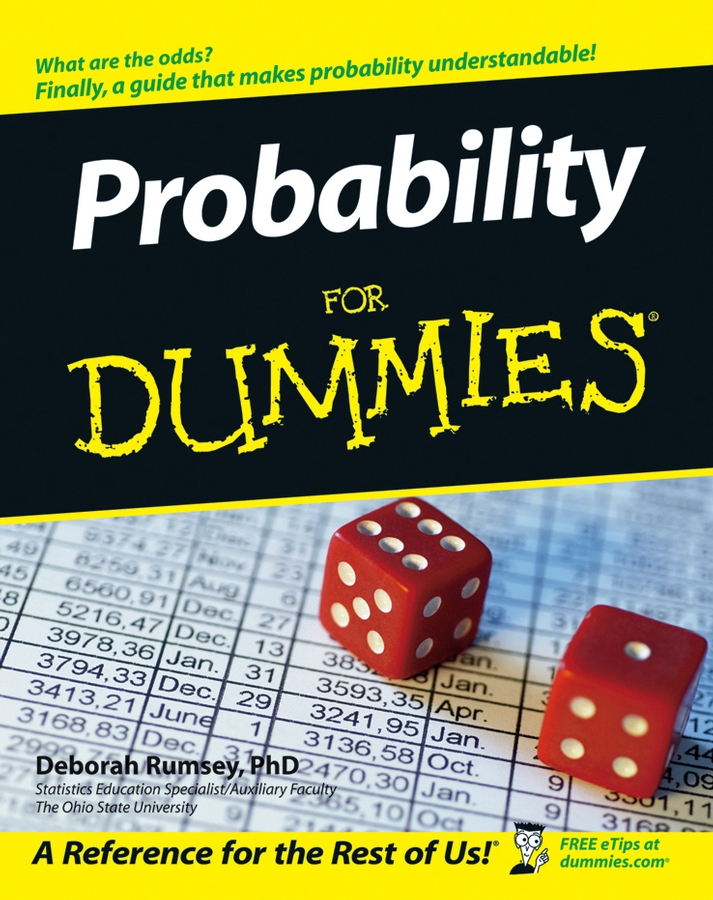Although the basic probability formula isn’t difficult, sometimes finding the numbers to plug into it can be tricky. One source of confusion is in counting the number of outcomes, both favorable and possible, such as when tossing coins and rolling dice.

## Tossing coins

When you flip a coin, you can generally get two possible outcomes: heads or tails. When you flip two coins at the same time — say, a penny and a nickel — you can get four possible outcomes: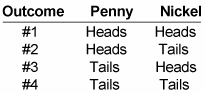When you flip three coins at the same time — say, a penny, a nickel, and a dime — eight outcomes are possible: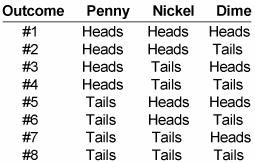Notice the pattern: Every time you add an additional coin, the number of possible outcomes doubles. So if you flip six coins, here’s how many possible outcomes you have:

2 2 2 2 2 2 = 64

The number of possible outcomes equals the number of outcomes per coin (2) raised to the number of coins (6): Mathematically, you have 26 = 64.

Here’s a handy formula for calculating the number of outcomes when you’re flipping, shaking, or rolling multiple coins, dice, or other objects at the same time:

Number of outcomes per objectNumber of objects

Suppose you want to find the probability that six tossed coins will all fall heads up. To do this, you want to build a fraction, and you already know that the denominator — the number of possible outcomes — is 64. Only one outcome is favorable, so the numerator is 1: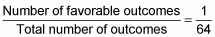So the probability that six tossed coins will all fall heads up is 1/64.

Here’s a more subtle question: What’s the probability that exactly five out of six tossed coins will all fall heads up? Again, you’re building a fraction, and you already know that the denominator is 64. To find the numerator (favorable outcomes), think about it this way: If the first coin falls tails up, then all the rest must fall heads up. If the second coin falls tails up, then again all the rest must fall heads up. This is true of all six coins, so you have six favorable outcomes: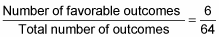Therefore, the probability that exactly five out of six coins will fall heads up is 6/64, which reduces to 3/32.

## Rolling dice

When you roll a single die, you can get six possible outcomes: 1, 2, 3, 4, 5, or 6. However, when you roll two dice, this number jumps to 36, as shown in the following figure.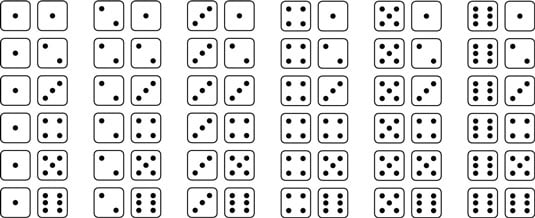Possible rolls for a pair of dice.
Every time you add an additional die, the number of possible outcomes is multiplied by 6. So if you roll four dice, here’s the number of possible outcomes:

64 = 6 6 6 6 = 1,296

Suppose you want to calculate the possibility of rolling four 6s. The probability is a fraction, and you already know that the denominator of this fraction is 1,296. In this case, only one outcome — all four dice coming up 6 — is favorable, so here’s how you build your fraction: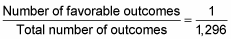So the probability that you’ll roll four 6s is 1/1,296 — a very small probability, indeed.

Here’s a more interesting question: What’s the probability that all four dice will come up 4, 5, or 6? Again, you’re building a fraction whose denominator is 1,296. To find the numerator, think about it this way: For the first die, there are three favorable outcomes (4, 5, or 6). For the first two dice, there are 3 3 = 9 favorable outcomes as shown here: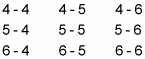For three dice, there are 3 3 3 = 27 favorable outcomes. So for all four dice, there are 3 3 3 3 = 81 favorable outcomes. So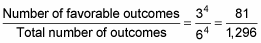Thus, the probability that all four dice will come up 4, 5, or 6 is 81/1,296. This fraction reduces to 1/16.